Introduction

In previous courses, when working with the exponent on a number the instructions were to do the exponential operation. For example, 24 should be rewritten as 16, because it is the simplest form of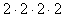. However with variables, exponents are used to simplify expressions, for example x7 is the simplified version of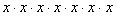.

Example 1 Simplify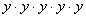.

This example is the multiplication of "y" 5 times, so it is simplified by writing: y5.

Example 2 Simplify (3a)(3a)(3a)(3a).

Step 1. Rearrange so that all numbers are grouped and all variables are grouped.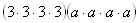Step 2. Multiply the numbers.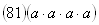Step 3. Use exponents to simplify the variables.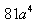*Note: Remember that when taking powers of negative numbers, you must take into account how many times the sign is being multiplied. Even powers of negative numbers create a positive number and odd powers of negative numbers create a negative number.

Listen